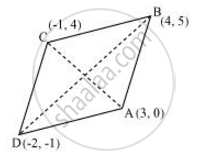# Find the area of a rhombus if its vertices are (3, 0), (4, 5), (− 1, 4) and (− 2, −1) taken in order. - Mathematics

Find the area of a rhombus if its vertices are (3, 0), (4, 5), (− 1, 4) and (− 2, −1) taken in order. [Hint: Area of a rhombus = 1/2 (product of its diagonals)]

#### SolutionLet (3, 0), (4, 5), (−1, 4) and (−2, −1) are the vertices A, B, C, D of a rhombus ABCD.

Length of diagonal AC =sqrt([3-(-1)]^2 + (0-4)^2)

Length of diagonal BD =sqrt([4-(-2)]^2+[5-(-1)]^2)

= sqrt(36+36) = 6sqrt2

Therefore area of rhombus ABCD = 1/2xx4sqrt2xx6sqrt2

= 24 square units

Concept: Section Formula
Is there an error in this question or solution?

#### APPEARS IN

NCERT Class 10 Maths
Chapter 7 Coordinate Geometry
Exercise 7.2 | Q 10 | Page 167